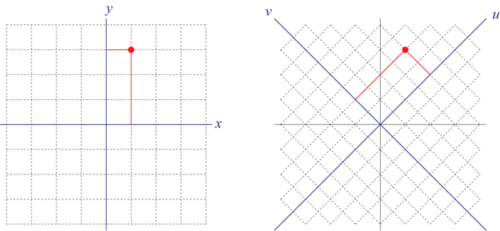# Giving thanks for the Fourier Transform

Sean Carroll in Preposterous Universe:

This year we give thanks for a technique that is central to both physics and mathematics: the Fourier transform. (We’ve previously given thanks for the Standard Model Lagrangian,Hubble’s Law, the Spin-Statistics Theorem, conservation of momentum, effective field theory, the error bar, gauge symmetry, and Landauer’s Principle.)

Let’s say you want to locate a point in space — for simplicity, on a two-dimensional plane. You could choose a coordinate system (x, y), and then specify the values of those coordinates to pick out your point: (x, y) = (1, 3).But someone else might want to locate the same point, but they want to use a different coordinate system. That’s fine; points are real, but coordinate systems are just convenient fictions. So your friend uses coordinates (u, v) instead of (x, y). Fortunately, you know the relationship between the two systems: in this case, it’s u = y+x, v = y-x. The new coordinates are rotated (and scaled) with respect to the old ones, and now the point is represented as (u, v) = (4, 2).

Fourier transforms are just a fancy version of changes of coordinates. The difference is that, instead of coordinates on a two-dimensional space, we’re talking about coordinates on an infinite-dimensional space: the space of all functions. (And for technical reasons, Fourier transforms naturally live in the world of complex functions, where the value of the function at any point is a complex number.)

More here.